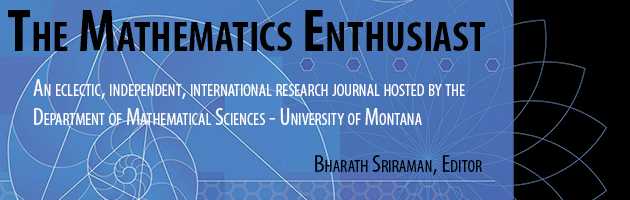•
•14

1-3

#### Abstract

This paper discusses Freudenthal's didactical phenomenology for the mathematical structures related to measurement. Freudenthal starts with the set G of all objects having the attribute of weight, an attribute that is to be measured. He proposes two operations, the first one among the measurements themselves, called “addition". Addition is interpreted in terms of the mental actions associated with measurement and, in the case of weights, it consists on the process of placing weights on one of the two dishes of a balance in order to balance them with a predetermined gauge, its fractions or multiples thereof, which are placed on the other. Their total weight is obtained by comparison with a predetermined gauge. The second operation, between weights and positive integers, allow weights to be amplified (by adding as many times as desired their weight measure). A related operation to amplification is that of contraction (by dividing the weight into as many equal parts as desired.) Freudenthal then extends his definitions in order to assign a meaning to the multiplication of a measure by, first, any positive rational number, and, second, by completeness, to any real number. Freudenthal's didactical phenomenology is based on the historical phenomenology of measurement, fractions, decimals and percentages. It is a central tenet of Realistic Mathematics Education (RME) that didactical phenomenologies of mathematical structures constitute proposals from which to make cognitively advantageous teaching sequences for the relevant mathematical structures. We discuss how Freudenthal's phenomenology of the mathematical structures for measurement gets translated into the mathematical models associated with the rational numbers. Finally, we make reference to a paradigmatic teaching unit which incorporates coherently Freudenthal's didactical phenomenology.

3

14

COinS